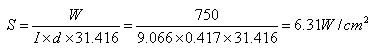## India Contact Information

BHUBAN CENTRE, P-512/3,PARNASHREE PALLY, KOLKATA – 700060, INDIA.

Tel: 0091 98304 19756
E-Mail: amitbanerjee@alloywire.com# Designing a Round Wire Element

Where:

 V Voltage (Volts) W Power (Watts) S Surface Area Loading (W/cm2) Rt Element Resistance at Operating Temperature (ohms) R Element Resistance at 20°C (ohms) F Temperature-Resistance Factor I Wire Length (m)

Example:

1.  To calculate the wire diameter and length required for a 750w/240v pencil bar fire element, operating at a maximum temperature of 1100°C, the total resistance of the element at operating temperature (Rt) will be: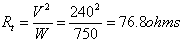2.  Using RW80 wire, the Temperature Resistance Factor (F) at 1100°C is 1.071 thus the total resistance of the element at 20°C (R) will be: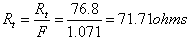3.  Knowing the dimensions of the pencil bar, the length of wire that may be wound round it may be estimated (see coiled or spiral elements).  Thus, the resistance required per metre of wire will be:

For example a length of wire of 9 metres –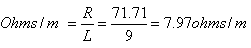4.  RW80 of wire diameter 0.417mm has a resistance per metre of 7.91 ohms/m (see stock sizes page) which is closest to 7.97 ohms/m.

5.  To verify the actual wire length (L):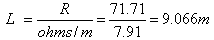A change in wire length may mean adding or subtracting turns (altering the pitch of the wire) to achieve the total resistance value required.